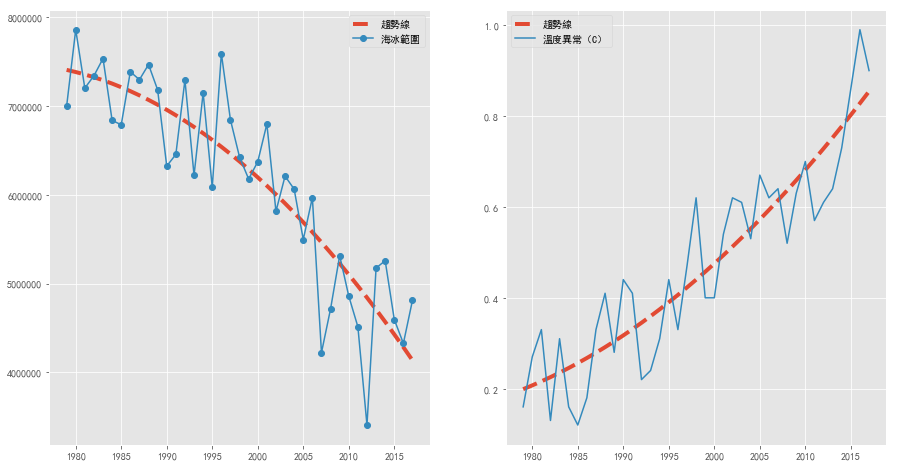## 發燒原因一：北極海冰減少問題¶

### 北極海冰範圍分析¶

#### 準備套件¶

In :
import pandas as pd


#### 抓取資料¶

In :
link_data_Arctic = "/Users/louishan/Desktop/nasa/Arctic-Sea-Ice-Minimum.csv"


In :
data_Arctic = pd.read_csv(link_data_Arctic, parse_dates=True)
Year_Arctic = data_Arctic["YR"]
Arctic = data_Arctic["ICE EXTENT"]

Out:
0    6998892.433
1    7862302.527
2    7203627.698
3    7342438.729
4    7537161.907
Name: ICE EXTENT, dtype: float64

#### 顯示資料¶

matplotlib 是幫助我們顯示資料出來的強大套件，透過 matplotlib 裡面的 pyplot 功能，可以讓我們看出北極的數據。

#### 準備繪圖套件¶

In :
from matplotlib import pyplot as plt

• 我們一樣給他一個小名叫做 plt 方便我們使用這個功能。

style 是 matplotlib 的 free style ，可以透過它來去選擇較清楚的背景。

In :
from matplotlib import style


#### 小技巧¶

matplotlib 有些小問題，就是無法顯示中文的 title，所以為了避免這個問題，我們使用這行程式告訴 Python 我們想要顯示中文。

In :
plt.rcParams['font.sans-serif'] = ['SimHei']


In :
from pylab import rcParams
rcParams['figure.figsize'] = 15, 8


In :
style.use('ggplot')


#### 顯示數據¶

In :
plt.plot(Year_Arctic, Arctic)

Out:
[<matplotlib.lines.Line2D at 0x10a29c6d8>]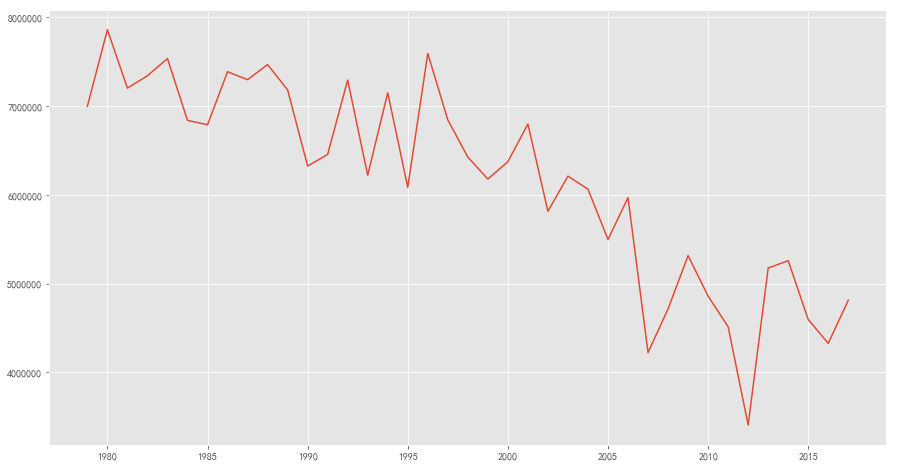### 北極海冰範圍在增加或減少？¶

#### 準備套件¶

In :
import numpy as np


#### 分析趨勢線¶

In :
get_Arctic_data = np.polyfit(Year_Arctic, Arctic, 2) #北極冰海年份和數據放入 polyfit 功能
Analysis_Arctic = np.poly1d(get_Arctic_data) #產生出線性方程式
Trendline_Arctic = Analysis_Arctic(Year_Arctic) #趨勢線


#### 顯示數據¶

In :
plt.plot(Year_Arctic, Trendline_Arctic, linestyle='--',linewidth= 4, label='趨勢線')
plt.plot(Year_Arctic, Arctic, linestyle='-', marker='o', label='海冰範圍')
plt.title('38 年北極海冰範圍 & 趨勢線 (1979 - 2017)')
plt.xlabel('年')
plt.ylabel('百萬平方公里')
plt.legend()

Out:
<matplotlib.legend.Legend at 0x10a5a0b38>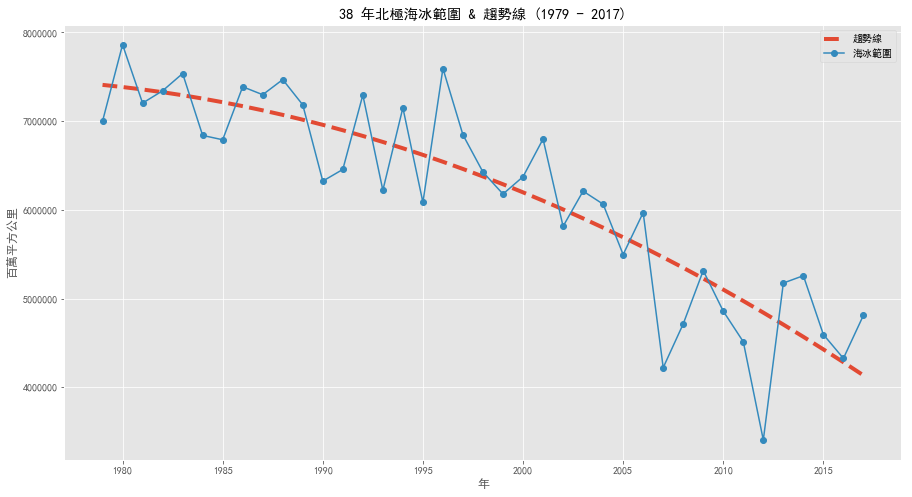### 異常溫度分析¶

#### 抓取資料¶

In :
link_data_Temperature = "/Users/louishan/Desktop/nasa/Global-Temperature.csv"


In :
data_Temperature = pd.read_csv(link_data_Temperature, parse_dates=True)
Year_Temperature = data_Temperature["YR"]
Temperature = data_Temperature["Temperature"]

Out:
0    0.16
1    0.27
2    0.33
3    0.13
4    0.31
Name: Temperature, dtype: float64

#### 顯示數#25818;¶

In :
plt.plot(Year_Temperature, Temperature)

Out:
[<matplotlib.lines.Line2D at 0x10a7bdeb8>]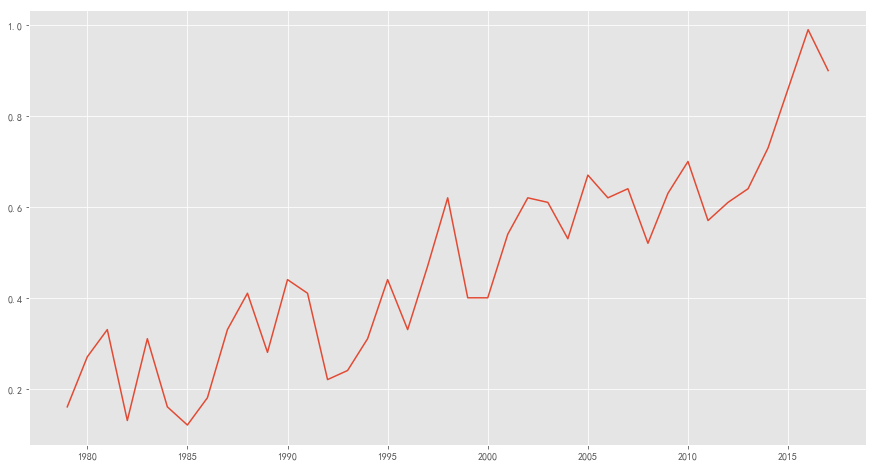#### 分析趨勢線¶

In :
get_Temperature_data = np.polyfit(Year_Temperature, Temperature, 2) #氣溫異常年份和數據放入 polyfit 功能
Analysis_Temperature = np.poly1d(get_Temperature_data) #產生出線性方程式
Trendline_Temperature = Analysis_Temperature(Year_Temperature) #趨勢線


#### 顯示數據¶

legend() 是負責顯示每條線代表意義的小框框。

In :
plt.plot(Year_Temperature, Trendline_Temperature, linestyle='--',linewidth= 4, label='趨勢線')
plt.plot(Year_Temperature, Temperature, linestyle='-', label='溫度異常（C）')
plt.title('38 年正負溫度異常 & 趨勢線 (1979 - 2017)')
plt.xlabel('年')
plt.ylabel('溫度異常（C）')
plt.legend()

Out:
<matplotlib.legend.Legend at 0x10ab5e828>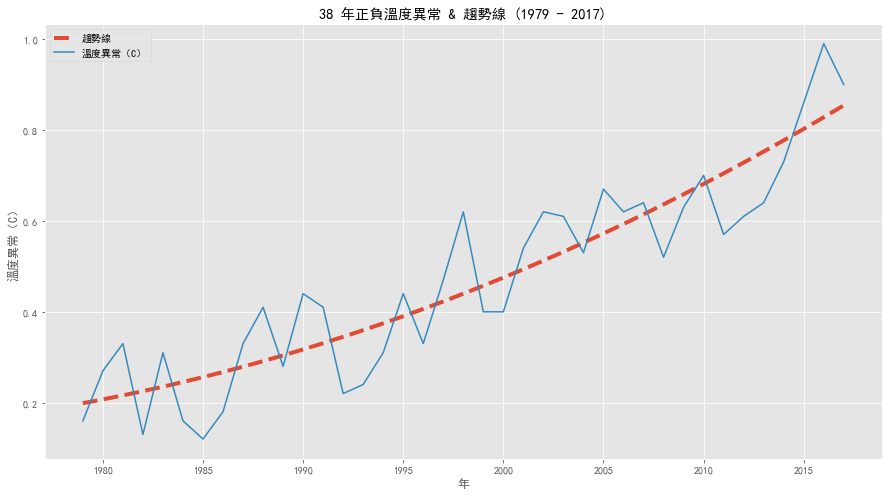### 診斷：觀察北極海冰 & 溫度異常圖¶

#### 顯示數據¶

left 和 right 變數簡顯易懂，在我們要 plot 出左邊的圖時，就使用 left 變數，right 變數則是讓我們可以顯示圖在右邊。

legend() 是負責顯示每條線代表意義的小框框，告訴 legend() 左右兩邊的圖裡都要顯示框框。

In :
fig = plt.figure()

left.plot(Year_Arctic, Trendline_Arctic, linestyle='--',linewidth= 4, label='趨勢線')
left.plot(Year_Arctic, Arctic, linestyle='-', marker='o', label='海冰範圍')
left.legend()

right.plot(Year_Temperature, Trendline_Temperature, linestyle='--',linewidth= 4, label='趨勢線')
right.plot(Year_Temperature, Temperature, linestyle='-', label='溫度異常（C）')

right.legend()

Out:
<matplotlib.legend.Legend at 0x10add15f8>